## Saturday, May 31, 2014

### Indiscernible properties

Max Black's argument against the principle of identity of indiscernibles (PII for short) contends that one could conceive of a plurality of objects with the exact same observable properties (for some notion of "observable" and "property"), and proposes the simple example of a universe exclusively composed of two exactly similar spheres some distance apart from each other: in this perfectly symmetrical setup there is no way to tell one sphere from the other except by arbitrarily picking one up, which selection can not be based on their observable properties; so, the spheres are different (there are two of them) but all their salient properties are the same, thus breaking PII.
Let us simplify the two-sphere example even further down to a universe with two points without any intrinsic physical property except their being at some distance of each other. In the 2P universe, formulated as a theory in second-order logic, there are no primitive properties (unary relations) to discuss about and only one primitive binary relation C defined as
C(x, y) := the distance from x to y is zero
(C stands for"colocated") satisfying the following axioms:
x C(x, x) (each point is colocated with itself),
xy ¬C(x, y), (for each point there is another one not colocated with it).
Now, if we accept the axiom scheme of comprehension, we have
xRy(RyC(x, y)),
which, in combination with the axioms for C, implies
xRy(Rx¬Ry),
that is, for each point x there is a property (namely that of being colocated with x) that x has and some other point y (which, in 2P, is tantamount to saying any other point) does not have. This seems to restitute PII.
To this reasoning Black could have retorted in (at least) two different ways:
• "Being colocated with x" is just a slightly disguised form of "being identical with x", which is trivially true of x itself and trivially false of any other entity, adding nothing to our initial assumption that 2P has a population of two.
• The reasoning involves two ill-defined properties, "being colocated with a" and "being colocated with b", where a and b are one point and the other, or the other way around: without any discernible feature to use, there is no way we can select a particular point out of the two we have in 2P.
The first objection we can easily dispose of: "being colocated with x" is a bound version (via comprehension) of C, which is a perfectly discernible, physical property of pairs of points —if I'm given two points, identical or not, I can certainly inspect whether they are colocated. The second counterargument is more interesting and, in my opinion, allows us to provide a clearer formulation of Black's thesis. The objection could be rephrased as: for the same reasons that naming the two points "a" and "b" requires that we can previously tell one point from the other, their univocally associated properties Ra and Rb are also indiscernible, even if they are different. We can formalize indiscernibility in the following way:
Definition. A second-order theory T (with or without equality) is said to have indiscernible entities if there exists a formula φ with two free variables such that
xy (φ(x, x) ∧ φ(y, y) ∧ ¬φ(x, y))   (1)
is a theorem of T and, for each model M of T and a, bM satisfying (1), the structure M' obtained by swapping a and b in all the relations of M is also a model of T.
If the set of primitive relations of T is finite, indiscernibility can be expressed as a statement in T itself (sketch of proof: augment (1) with a conjunction of terms expressing swappability of x and y with respect to each position of each primitive relation of T). If T has equality, the statement
xy (φ(x, x) ∧ φ(y, y) ∧ ¬φ(x, y) ∧ xy)   (2)
is a theorem of T (proof trivial). 2P has indiscernible entities (proof trivial).
In conclusion, a formal interpretation of PII resists Black's attacks, but the following, stricter version of the principle:
If two entities have the same discernible properties, they are identical,
is false, and probably what Black had originally in mind.

## Friday, May 16, 2014

### The perfect shape

A liquid container designed to keep its content cool as long as possible would be spherical in shape, since the sphere is the closed surface that has the minimum area to enclosed volume ratio, thus minimizing convection heating. When the container is also meant to drink from, the sphere is no longer the optimum shape for coolness (leaving aside the fact that one should have to pierce it to access the liquid) because the surface of the remaining liquid changes as the container is being emptied.
A volume V of liquid at temperature T inside a glass is heated by convection on two different surfaces, one in direct contact with the environment and the other as enclosed by the glass wall.
These two surfaces contribute to the increase in temperature, given by Newton's law of cooling (here heating):
dT = (1/cvV)(hopen Aopen + hclosed Aclosed)(Tenv - T) dt,
where cv is the volumetric heat capacity of the liquid, hopen and hclosed the overall heat transfer coefficients of the open and closed interfaces, respectively, and Tenv the temperature of the environment. If the liquid is drunk at a constant rate of ϕ units of volume per unit of time, we have
dT = (1/cvϕV)(hopen Aopen + hclosed Aclosed)(T - Tenv) dV,
with Aopen and Aclosed varying as functions of V; this differential equation implicitly gives T as a function of (Aopen, Aclosed, V), although its analytical solution is only feasible for the simplest shapes. We define the optimum container as that for which the average temperature of the liquid during consumption
Tavg = [Vinit,0]TdV
is minimum.
Let us calculate this shape numerically. Candidate glasses are restricted to surfaces of revolution generated by nonnegative functions y = f(x) between 0 and some maximum height h.
We fix the rest of parameters: the contained liquid is one liter of water (or beer, for that matter) brought out of the fridge at 5 ºC and drunk in 3 minutes in a very hot summer afternoon at 40 ºC; the glass wall (made of, well, glass) is set to be 3 mm thick. All of this gives us:
Vinit = 1,000 cm3,
ϕ = 1,000/180 cm3/s,
Tinit = 5 ºC,
Tenv = 40 ºC,
cv = 4.1796 J/cm3/K,
hwater = 50 W/m2/K,
hair = 100 W/m2/K,
θglass = 3 mm,
kglass0.85 W/m/K,
hopen = 1/((1/hwater)+(1/hair)) = 33.3 W/m2/K,
hclosed = 1/((1/hwater)+(θglass/kglass)+(1/hair)) = 29.8 W/m2/K.
We will use a genetic algorithm to solve the problem:
• Candidate solutions are codified as arrays with the values f(0), f(0.5 cm), ... , f(50 cm) (that is, the maximum height of the glass is h = 50 cm, much higher than really needed —excess height will simply manifest as a tiny column of radius ≈ 0).
• The initial pool of candidates is populated with values from random Hermite interpolation polynomials of degree 3 normalized so that the enclosed volume is Vinit.
• Individual fitness is simply its Tavg calculated figure: the lower Tavg the fitter the solution.
• At each generation, the fittest 25% of the pool is kept and the rest replaced by random breeding of the former. Crossover of f0 and f1 is implemented by randomly selecting two cutoff points c1 and c2, putting f as:
• f(x) = f0(x) for x < c1,
• f(x) = f0(x)·(c2- x)/(c2- c1) + f1(x)·(x - c1)/(c2- c1) for c1x < c2,
• f(x) = f1(x) for xc2,
and normalizing f.
• Bred individuals are mutated (before normalization) by multiplying some of their values (mutation rate = 1%) by a random factor in the range [0,2).
The calculation program has been implemented in C++ (Boost, and in particular Boost.Units, is used). Trials with different initial populations and algorithm parameters (mutation rates, pool sizes and so on) yield basically the same result, which is depicted in the figure:Optimum glass profile.
The area of this glass is 537 cm2 and its associated average temp Tavg is 6.40 ºC (quite cool!). As predicted, the excess height from ~25 cm up shows as a tiny filament whose actual capacity is totally negligible: trimming the glass cap off at a height of 18.5 cm produces a more practical glass with an opening diameter of 4.2 cm and almost the same performance (we only dropped 2% of the initial content and the temperature of the liquid at that point, 3.6 seconds after beginning to drink, is only slightly higher than Tinit). The glass thus obtained is, perhaps surprisingly, very reminiscent of real-life glasses: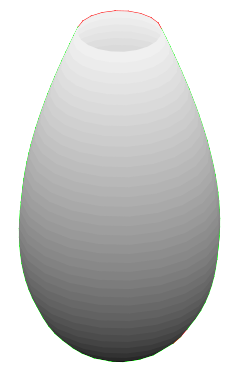Optimum glass trimmed at 18.5 cm.
In general, trimming the optimum glass f(x) for a given Tinit at height hcut produces the optimum solution of the problem for Vinit = V(height = hcut), Tinit = T(height = hcut) with the additional constraint that the opening of the glass is precisely 2f(hcut): we can then play with this property to obtain solutions to the modified problem
minimize Tavg while maintaining a given opening diameter δ
by starting with Vinit+ ΔV and Tinit -  ΔT and adjusting ΔV and ΔT until the volume and temperature at the desired opening hit Vinit and Tinit simultaneously (experimenting with this is left as an exercise for the reader).
Parameter sensitivity
An informal analysis reveals that the optimum glass shape is quite independent of changes in most problem parameters. In particular:
• Tinit does not affect significantly the solution (as long as Tinit < Tenv).
• Changing Vinit simply produces a scaled version of the same shape.
• Decreasing ϕ (that is, taking longer to drink the beer up) yields only slightly different shapes, with a wider lower segment.
The one aspect that truly changes the shape is the hopen/hclosed ratio. As this increases, the optimum glass gets thinner at the base and longer, approaching a cylinder as hopen/hclosed → ∞. This has practical implications: for instance, making the beer grow a thick foam topping reduces hopen, which allows for shorter, wider, rounder glasses.
Postscript
Some readers have asked why we haven't used calculus of variations to solve the problem. In its classical one-dimensional formulation (Euler-Lagrange equation), this calculus provides tools for finding the stationary values of a functional J(f) defined as
J(f) = [a,b] L(f(x), f'(x), x) dx,
for some function L : ℝ3 → ℝ with appropriate smoothness properties. The important aspect to note here is that L must depend on the point values of f and f' at x alone: by contrast, in our case the integrand T(V) is a function of hopen Aopen(v) + hclosed Aclosed(v) for all values of v in [V, Vinit], so the conditions for the application of Euler-Lagrange equation do not hold.

## Wednesday, May 7, 2014

### Fast polymorphic collections with devirtualization

The poly_collection template class we implemented for fast handling of polymorphic objects can be potentially speeded up in the case where some of the derived classes it manages are known to the compiler at the point of template instantiation. Let us use this auxiliary template class:
```template<class Derived>
class poly_collection_static_segment
{
public:
void insert(const Derived& x)
{
store.push_back(x);
}

template<typename F>
void for_each(F& f)
{
std::for_each(store.begin(),store.end(),f);
}

template<typename F>
void for_each(F& f)const
{
std::for_each(store.begin(),store.end(),f);
}

private:
std::vector<Derived> store;
};
```
We can add the ability for poly_collection to accept a variable number of derived class types:
```template<class Base,class ...Derivedn>
class poly_collection
{
...
};
```
so that it includes poly_collection_static_segment<Derivedi> components taking care of objects whose types concide exactly with those specified, whereas for the rest the default dynamic handler is still used.
What does this gain us? Consider the example:
```class base
{
virtual void do_stuff()=0;
};

class derived1:public base{...};
...
poly_collection<base,derived1> c;
c.insert(derived1(0));
...
c.for_each([](base& x){
x.do_stuff();
});
```
For the segment handling derived1, the code in for_each resolves to:
```std::for_each(store.begin(),store.end(),[](base& x){
x.do_stuff();
});
```
where store is of type std::vector<derived1>: so, the compiler can know that x.do_stuff() is invoked on objects with exact type derived1 and hence omit vtable lookup and even, if the definition of derived1::do_stuff is available at instantiation time, inline the call itself.
Now, the fact that the compiler can apply these optimizations does not mean it necessarily will do so: the static analysis needed to determine the optimization opportunity is certainly not trivial. There are a number of techniques we can implement to help the compiler in the process:
1. Make derived1::do_stuff final.
2. Use a polymorphic lambda [](auto& x){x.do_stuff();} to omit the cast from derived1& to base&.
3. Do both 1 and 2.
I have written a test program (Boost required) that implements this new version of poly_collection and measures its for_each performance for the following scenarios:
1. poly_collection<base> (baseline)
2. poly_collection<base,derived1>
3. poly_collection<base,derived1,derived2>
4. poly_collection<base,derived1,derived2,derived3>
5. poly_collection<base,final_derived1>
6. poly_collection<base,final_derived1,final_derived2>
7. poly_collection<
base,final_derived1,final_derived2,final_derived3
>
8. same as 1, with a polymorphic functor
9. same as 2, with a polymorphic functor
10. same as 3, with a polymorphic functor
11. same as 4, with a polymorphic functor
12. same as 5, with a polymorphic functor
13. same as 6, with a polymorphic functor
14. same as 7, with a polymorphic functor
with containers of several sizes ranging from n = 1,000 to 107 elements (except where noted, results do not vary significantly across sizes). Values are the averages of execution times / number of elements for each scenario, normalized to the baseline = 1.
MSVC 2012
Microsoft Visual Studio 2012 using default release mode settings on a Windows box with an Intel Core i5-2520M CPU @2.50GHz.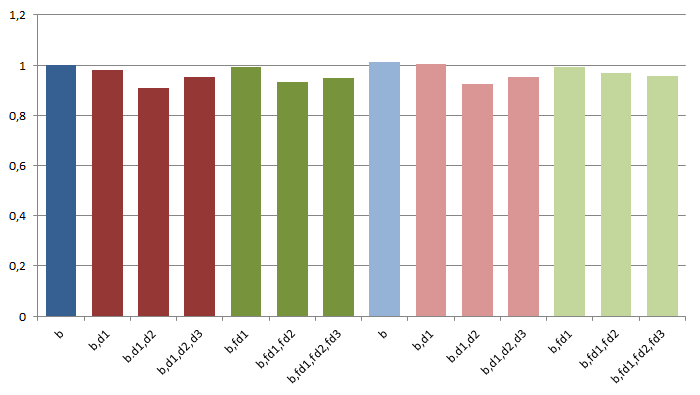Normalized execution times / number of elements.
Differences in performance are not significative and can be labeled as mostly noise: in fact, a visual inspection of the generated assembly code reveals that virtual calls are not optimized ever.
MSVC 2013
Results by Lars Schouw: MSVC 2013 with default release settings on an box with an Intel Core i7-920 @2.67GHz.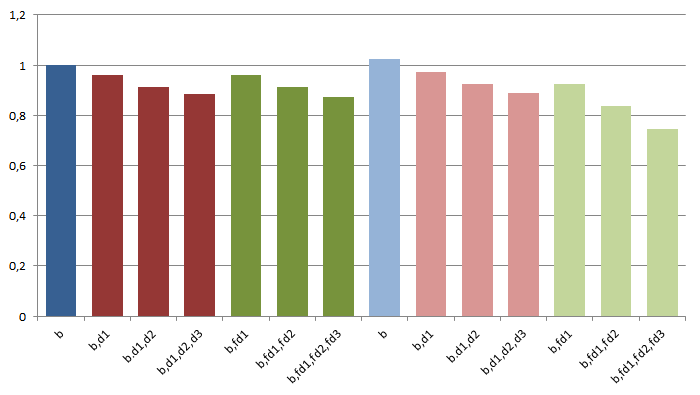Normalized execution times / number of elements.
Unlike with MSVC 2012, here there is a modest but consistent improvement in performance as more derived classes are statically specified. This is probably due to tighter traversal loops rather than devirtualization, except when a polymorphic lambda is combined with final virtual functions, where reductions of up to 25% seem to indicate that inlining is taking place.
Clang on Arch Linux
Results by Theodoros Theodoridis: Clang 3.4 with settings -std=c++11 -O3, Arch Linux x64 with an Intel Core i7-950 @3.07GHz.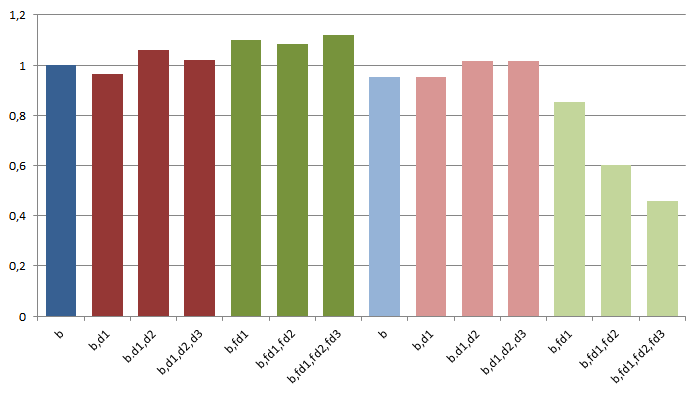Normalized execution times / number of elements.
In most cases, specifying derived classes has no or a detrimental effect on performance (the reason for the degradation is not clear to me). For polymorphic lambda + final virtual functions, however, reductions in execution times are impressively large.
There is an odd effect that the aggregate figures of the graph do not show: in the last two scenarios, performance results when n = 107 are still much better than the baseline but comparatively worse than for other container sizes.
Clang on Ubuntu 64
Results by plash: Compiler Clang 3.5.0 with settings -std=c++11 -stdlib=libc++ -lc++ -O3, system Ubuntu 13.04 x86_64 with an AMD Phenom II.Normalized execution times / number of elements.
Specification of derived classes definitely improves execution times, although the resulting performance patterns and underlying factors are not easily interpretable. For polymorphic lambda + final virtual functions we have again massive reductions in execution time. Similarly to the case with Clang 3.4, we have comparatively poorer performance for  n = 10, although here the effect is seen across all scenarios.
GCC on Arch Linux
Results by Theodoros Theodoridis: GCC 4.9.0 with settings -std=c++11 -O3, Arch Linux x64 with an Intel Core i7-950 @3.07GHz.Normalized execution times / number of elements.
For this compiler, passing a monomorphic or a polymorpic lambda to for_each makes all the difference, with the latter case achieving reductions in execution time of up to 60%. I do not have an explanation as to why using final virtual functions improves on the non-final case: in both situations inlining seems to be in effect, which should render final qualification irrelevant. Like with Clang, some cases of relative degradation when  n = 107 are also seen for this compiler, here mostly concentrated on the last two scenarios.
(Update Aug 17, 2014) GCC 5 on Linux
Jan Hubička is working on integrating devirtualization capabilities in GCC, and the results on this area are improving accordingly. He ran the test benchmark against GCC mainline (as of Aug 15, 2014) with settings -O3 on a Linux machine with an Intel i5-4300U processor.Normalized execution times / number of elements.
GCC's speculative devirtualization analysis is able to take full advantage of the optimization opportunities offered by static knowledge of the types involved, even to the point of rendering final decoration superfluous.
Conclusions
Allowing for the specification of a list of statically known derived class types as part of a poly_collection instantiation opens up possibilities for internal performance optimizations that do not impact the usage interface of this template class. The actual improvements achieved depend very much on the compiler being used, but in general are greater when passing polymorphic functors (such as generic lambdas) to for_each and qualifying virtual functions as final where possible.
Postscript: The observant reader might have noticed that poly_collection_static_segment<Derivedi> does not explicitly require that Derivedi be an actual derived class from Base, and in fact one can pass any type as long as we use a polymorphic functor with for_each that accepts it:
```poly_collection<std::string,int,char> c;
c.insert(std::string("hello"));
c.insert(0);
c.insert('a');
c.for_each([](auto& x){
std::cout<<x<<"\n";
});

Output:
hello
0
a
```
This comes more as a (easily fixable) happy accident than from a conscious design decision, but hints at an area of exploration away from the original purpose of poly_collection, focused on handling heterogeneous classes via visitor patterns in a manner reminiscent of the techniques used in Boost.Variant.
Update Jan 17, 2015
Fixed a serious bug in the code, that, fortunately, doesn't affect the test results.

## Sunday, May 4, 2014

### Fast polymorphic collections

In C++, instances of classes derived from an abstract class are typically heap-allocated and managed through (smart) pointers because their exact types (and hence their sizes) are not known until run time —strictly speaking, the type of each object is known at the point of creation but then abstracted away or erased when passed along to the location of the program where it is stored and used. For this very reason, such objects can't be stored in STL collections directly but rather through an indirection. For instance, this is how the layout of a std::vector<std::unique_ptr<base>> could look like for some abstract base class:
Sometimes we are not interested in the particular order the objects are stored in as we only want to keep them together to interact with them in a uniform manner:
```struct base
{
virtual int do_stuff(int x)=0;
};
...
using base_pointer=std::unique_ptr<base>;
std::vector<base_pointer> c;
...
std::for_each(c.begin(),c.end(),[](base_pointer& p){
p->do_stuff(1);
});
```
When c.size() is large the resulting performance is impacted by two negative factors:
• Heap allocated objects might be scattered throughout the memory space, incurring many cache misses during traversal;
• Modern architectures with branch prediction can optimize away the vtable lookup cost associated to virtual calls when these calls successively resolve to the same function, but this is not the case if objects of distinct derived classes are interspersed along the vector.
Let us explore a different structure where objects of the same derived class are held together in segments of contiguous memory:
Here, objects are appended to their associated memory segment on insertion time, for which we need to know their exact type at that point. The structure used to determine which segment each object is inserted into is then just a std::map of std::type_index's to segment handling objects. This is a minimal interface for such a data structure:
```template<class Base>
class poly_collection
{
public:
// only available if Derived is derived from Base
template<class Derived>
void insert(Derived& x);

template<typename F>
F for_each(F f);

template<typename F>
F for_each(F f)const;
};
```
Profiling
A testing program is provided that implements poly_collection and compares the performance of poly_collection<base> with respect to std::vector<std::unique_ptr<base>> for the following scenario:
```struct base
{
virtual int f(int x)const=0;
};

struct derived1:base
{
derived1(int n):n(n){}
virtual int f(int x)const{return x*n;}

int n;
};

struct derived2:base
{
derived2(int n):n(n){}
virtual int f(int x)const{return x+n;}

int unused,n;
};

struct derived3:base
{
derived3(int n):n(n){}
virtual int f(int x)const{return x-n;}

int unused,n;
};

template<typename Collection>
int run_for_each(const Collection& c)
{
int res=0;
c.for_each([&res](const base& x){
res+=x.f(1);
});
return res;
}
```
where the containers are filled with an assortment of n = 1,000 to 107 objects of types derived1, derived2 and derived3 and then randomly shuffled to reflect the memory status of a program where objects have heterogeneous lifetimes.
MSVC on Windows
Test built with Microsoft Visual Studio 2012 using default release mode settings and run on a Windows box with an Intel Core i5-2520M CPU @2.50GHz. Depicted values are microseconds/n, where n is the number of elements in the container.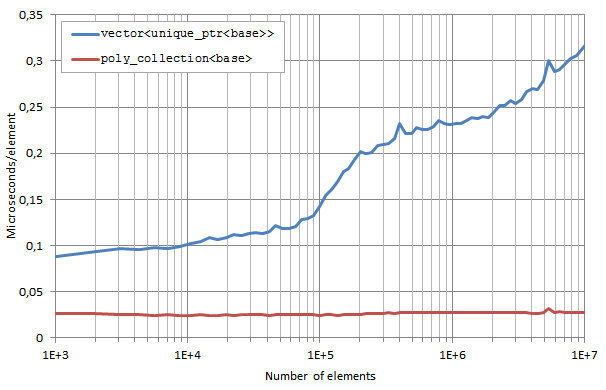for_each execution times / number of elements.
poly_collection behaves excellently and is virtually not affected by the size of the container. For n < 105, the differences in performance between poly_collection and a std::vector of std::unique_ptrs are due to worse virtual call branch prediction in the latter case; when n > 105, massive cache misses are added to the first degrading factor.
For MSVC 2013 on an box with an Intel Core i7-920 @2.67GHz, results (provided by Lars Schouw) are similar, though the sharp steep in execution times for std::vector<std::unique_ptr<base>> is shifted from ~105 to ~3·105 due to the larger memory cache of the i7-920 processor.for_each execution times / number of elements.
Clang on Ubuntu 64
Results provided by plash: Compiler Clang 3.5.0 with settings -std=c++11 -stdlib=libc++ -lc++ -O3, system Ubuntu 13.04 x86_64 with an AMD Phenom II X6 1090T.for_each execution times / number of elements.
Results are even more striking here: while for small values of n poly_collection is only 2x faster than a std::vector of std::unique_ptrs (3x for MSVC), performance ratio is 25x for n =  107 (10-12x for MSVC).
GCC on Arch Linux
Results provided by Theodoros Theodoridis: Compiler GCC 4.9.0 with settings -std=c++11 -O3, system Arch Linux x64 with an Intel Core i7-950 @3.07GHz.for_each execution times / number of elements.
Other environments
Request for help: if you can build (with release settings) and run the test program on some other environment/compiler and post the results back I'd be happy to include them in the entry.
Conclusions
Modern architectures, heavily accelerated by CPU memory caches, penalize typical patterns of C++ run-time polymorphism based on individual heap allocation of many heteogeneous objects implementing a given abstract interface. This can be improved dramatically by using containers that gather objects of the same concrete type in segments of contiguous memory, if this type is known at the point of insertion: poly_collection provides the foundational ideas for such a container. Note that poly_collection requires the classes dealt with to be CopyConstructible and MoveInsertable, which involve operations typically not used in classic patterns of C++ dynamic polymorphism.
There is still margin for improvement: if some of the concrete types deriving from base are known at container definition time, we can use this knowledge so that poly_collection benefits from devirtualization of these types; this we will explore in a later entry.
Update Jan 17, 2015
Fixed a serious bug in the code, that, fortunately, doesn't affect the test results.